# What is a Linear Foot and How to Calculate It

Sep 25, 20234 min read

## What is a linear foot?

A linear foot is 12 inches in a straight line. Using the term “linear” indicates that you are measuring in a straight line. Anytime you measure something in a straight line in the United States, you’re measuring how long that thing is in linear feet.

Linear feet differ from square feet and cubic feet, though. And whether you’re measuring your yard, your moving truck, or the length of your fence, it’s important to know how and when to use these different measurements. Taking proper measurements is also an essential part of filling out a home inventory for your moving company.

Keep reading to learn all there is to know about linear feet, square feet, and cubic feet. Along the way, we’ll also throw in a few conversion charts to help with all the math you’ll need to correctly measure your stuff.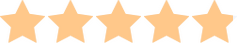4.9

### Our top-rated mover

International Van Lines’ comprehensive list of moving services, worldwide availability, and unparalleled customer service make it one of the best options for any move.

## The full picture

### How to measure and calculate a linear foot

If you have a ruler or a measuring tape with markings in one-inch increments, you’re ready to measure anything in linear feet. Remember, a linear foot is 12 inches in a straight line.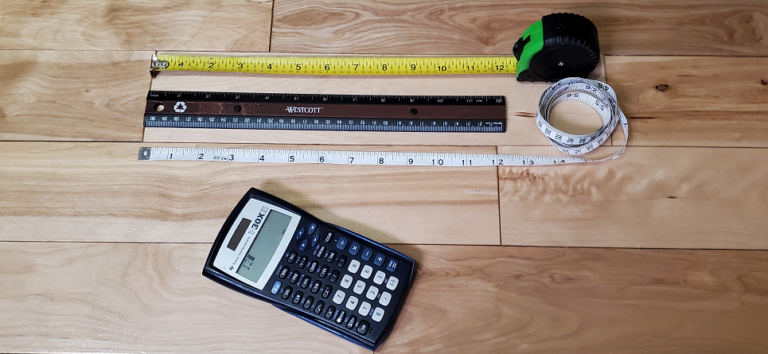A linear foot measured on a ruler and two measuring tapes.

If you measure something and it’s 12 inches long, it’s one linear foot in length. If it’s 24 inches long, that’s two linear feet, etc. The nice thing is that most tape measures have markings for feet as well as inches, so you may not even need to do the calculations yourself.

But just in case your measuring tape doesn’t have markings for feet or you’re using a 12-inch ruler for all your measurements, here’s a handy conversion chart to make the math easy:

#### Inches to linear feet conversion chart

12 inches 24 inches Inches Linear feet 1 linear foot 2 linear feet 3 linear feet 4 linear feet 5 linear feet 6 linear feet

Of course, not all measurements can be nicely divided by 12. For example, if your dining table is 63 inches long, you won’t be able to write down its dimensions using only feet.

For situations like this, find the closest number divisible by 12 to the object’s length, calculate how many feet that is and write it down, and then write down how many inches are leftover. The length of the table from our example would be written as “5 feet 3 inches” or “5′3″.”

#### When to measure in linear feet

Use linear feet to measure anything in a straight line. If you need to know the length of a rug, the width of a bookcase, or the height of your child, linear feet will do the trick.

 Company Company type Move.org rating Packing & loading services Pricing Discounts Learn more Professional mover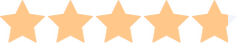4.8Yes \$\$\$10% Move.org discount, military personnel, seniors Get a Quote Professional mover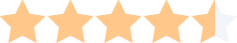4.5Yes \$\$\$None Get a Quote Professional mover4.8Yes \$\$\$Seniors Get a Quote Moving container company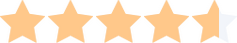4.6No \$\$10% Move.org discount, military personnel Get a Quote Moving container company4.5No \$\$Move.org readers, military personnel Get a Quote

### What is square footage?

Square footage is used to measure the area of a flat surface. It can tell you how big your yard is, how much floor space your bed will take up, and how much wall space you’ll need for all your concert posters.

When you calculate square footage, you actually use linear feet as part of the math.

To begin finding the square footage of an object or space, measure its length in linear feet. Then, measure it in one other direction. You only need to take two measurements to find square footage, but which measurements you should take depend on what you’re measuring.

To find a wall’s square footage, measure its length and its height. Similarly, to know how much wall space a piece of decor or furniture will take up, measure its length and its height. To find the square footage of your kitchen floor or learn how much floor space something will take up, measure its width and its length.

Once you have these two measurements, multiply them by each other. The product is the object’s or space’s square footage. For example, if your garage is 10 feet wide and 16 feet long, the area of your garage floor is 160 square feet.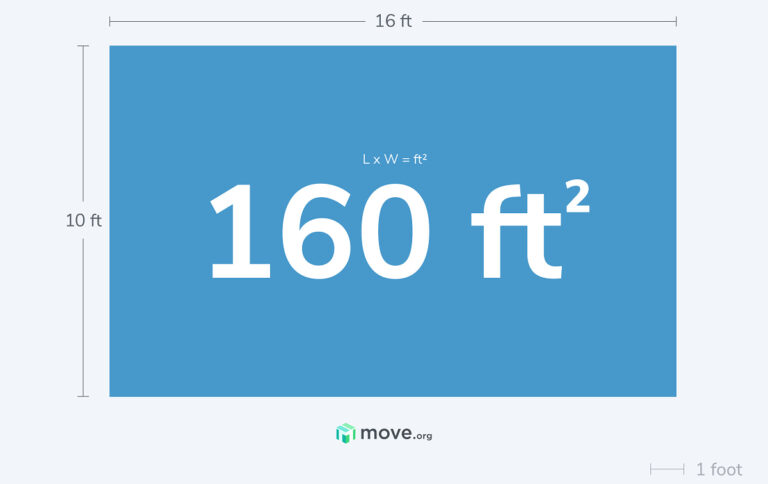This is an example of how to calculate the square footage of a flat surface that's 10 ft. long x 16 ft. wide.

The math for calculating square feet can be a bit trickier than measuring linear feet, so you’ll probably need a calculator, but here’s a conversion chart that illustrates how to do it:

#### Linear feet to square feet conversion chart

5 feet long x 10 feet tall 2 feet wide x 7 feet long Dimensions in linear feet Square footage 50 square feet 14 square feet 500 square feet 1,680 square feet

#### When to measure in square feet

Use square feet whenever you need to know the total area of a flat surface or the footprint of a piece of furniture. Square footage can tell you how big a rug is, how much floor space your coffee table will take up in your living room, or how large your patio is.What if an object or space is round?

Calculating the square footage and cubic footage of round objects requires more complex math, so you should only really do it with a calculator. Here are some handy calculators that can find the square footage and cubic footage of round objects for you.

### What is cubic footage?

Cubic footage is used to calculate a container’s volume and how much room an object will occupy in a container. In short, it can tell you how much stuff you can fit into things like boxes, cabinets, rooms, moving trucks, and storage units.

To find the cubic footage of a space or an object, you’ll once again start by measuring linear footage. This time, though, you’ll measure in all three directions: length, width, and height.

Once you have all three dimensions, you’ll multiply them together. The product is the cubic footage. For example, if a moving truck’s cargo compartment is 10 feet long, 6 feet wide, and 6 feet high, the compartment is 360 cubic feet in volume.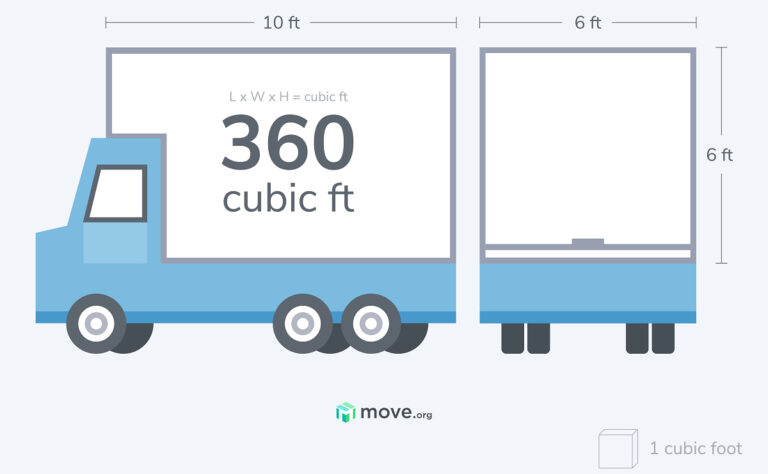This is an example of how to calculate the capacity of a moving truck's cargo area in cubic footage.

Now, the numbers involved in cubic feet get pretty big, and the math can be a little too tricky to quickly do in your head, so use a calculator when trying to find an object's cubic footage. To illustrate exactly how to do this, here are a few examples:

#### Linear feet to cubic feet conversion chart

2 feet long x 5 feet wide x 10 feet tall 7 feet long x 10 feet wide x 6 feet tall Dimensions in linear feet Cubic footage 100 cubic feet 420 cubic feet 750 cubic feet

#### When to measure in cubic feet

Measure in cubic footage whenever you need to know how much a container can hold or how much space an object will take up inside a container.

For example, finding the cubic footage of your moving truck, storage unit, or moving and storage container is the only way to know exactly how much stuff you can put in them. Of course, you’ll also want to know the cubic footage of everything you plan to put inside these containers to ensure it’ll all fit.

## FAQs about linear feet and other measurements

A linear foot is 12 inches in a straight line, which is why it is called a “linear” foot.

A square foot is a flat area that measures 12 inches long and 12 inches wide, a perfect square. The entire area of a square foot is 144 square inches.

A cubic foot is a three-dimensional space that measures 12 inches long, 12 inches high, and 12 inches wide, like a cube. The volume of a cubic foot is 1,728 cubic inches.

There are three linear feet in a linear yard, so a yard is 36 inches long.

A meter is 3.28 feet—about 39 inches—in length. This makes a meter only slightly larger than a yard. For quick conversion between feet and meters, use this calculator.

## Recommended resources

Looking up measuring info because you’re planning a move and need to know how big your stuff is? These resources can help you plan and prepare for moving day:Written by
Joe Roberts
Joe Roberts is a professional writer with a degree in writing studies and over four years of copywriting experience. He previously worked at Overstock.com, where he wrote about furniture, home decor, and moving. Joe has moved all over Utah, so he knows his way around a moving truck—and he spends his time (and money) expanding his personal library so it will be even heavier next time he moves.